HOME

BACK(to QQM)

# One Dimensional Free Particle Dirac Equation

## Dirac Equation

We start with the following one-dimensional form of the free particle Dirac Equation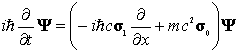(1)

The wave functionis a complex two component vector:(2)

The probability density is: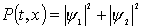(3)

(That is to say the probability of finding the particle in a space time region is)
Thecan be represented as complex matrices with the conditions: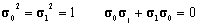(4)

Amongst numerous possibilities we will use: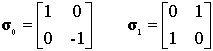(5)

The equation can be written out as: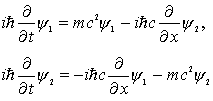(6)

## Conservation of Probability

It is easy to show from the differential  equation that: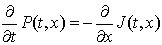(7)

Where: the current J(t,x) is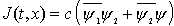(8)

## Plane Wave Solutions

We categorize two types of plane wave solutions, according to positive or negative energy: Let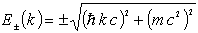(9)

(Throughout this article  the square root function is taken to be the branch with positive real part)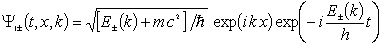(10)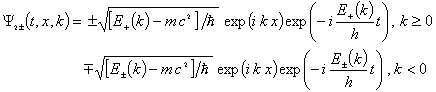(11)

## Energy and momentum operators

Just as in non-relativistic theory the energy and momentum operators are: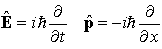(12)

The eigenvalue of these operators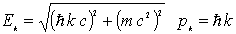(13)

are related by the ‘classical’ relativistic expression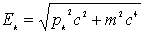(14)

In Dirac theory the negative branch of the square root leads to negative energy solutions

## Construct a complete and orthogonal  set of plane waves

It is easy to verify (but follow the rule on the square root branch strictly and carefully) that(15)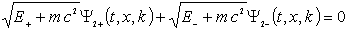(16)

We can now construct various wave packets with the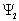component initially zero and with arbitrary initial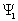component. For example this wave packet starts with the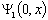component a real gaussian and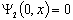.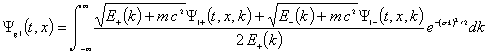(17)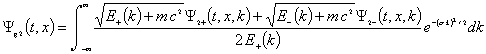(18)

## Animation of the wave packet formed from positive and negative energy solutions:

(Move the mouse cursor over the graphs to start the animation. you must enable GIF animation and JavaScript in your browser)
First a wide wave packet: the width parameterOn the animation you will notice an oscillation imposed on the overall spreading of the packet. This oscillation is sometimes referred to as zitterbewegund (jitter-motionthe oscillation frequency is mc2/h

## Narrow wave packets formed from negative and positive solutions

If the initial width of the packet is smaller than the wavelength of this oscillation than the wave packet evolution is surprisingly different: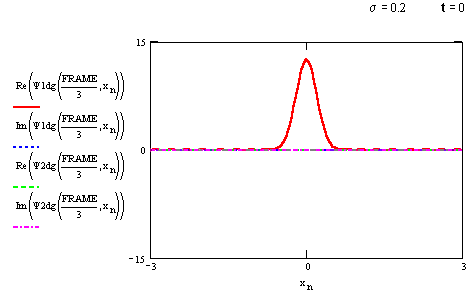## Solutions with positive energy waves

The negative energy solutions are unphysical in nature; it would be nice to form gaussian wave packets using only the positive energy solutions.  This we can do, but we can’t set the both components to arbitrary initial conditions: there aren’t enough degrees of freedom.

We can however, start out  with zero current and  real gaussian probability distribution as follows. First,note that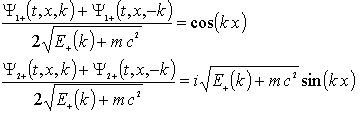.                                  (19)

The first expression is real the second expression is imaginary so the current is zero(see eq8). We form a wave packet that is gaussian like initially with zero current: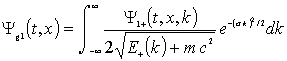(20)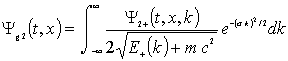(21)

Again, animations, first with a relatively wide wave packet withThe main qualitative difference here is that the zitterbewegund is not visible in the probability distribution.

Again the narrow packets split up and travel at about the speed of light: The physical reason for this behavior lies in the uncertainty principle. The extreme localization to a distance smaller than the Compton wavelength imparts large momentum. to the particle

If you have MathCad 8 or higher, you may wish to examine the file that generated these graphs(about 60Kbyte): Dirac1Dwp.mcd

HOME

BACK(to QQM)